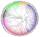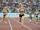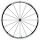# Circle simple

Circumference of a circle is 6.28. What is the area of the circle?

Result

S =  3.138

#### Solution:Leave us a comment of example and its solution (i.e. if it is still somewhat unclear...):

Showing 0 comments:Be the first to comment!## Next similar examples:

1. Circle - simpleThe circumference of a circle is 930 mm. How long in mm is its diameter?
2. DiameterThe diameter of a circular plot is 14 dm. Find the circumference and area.
3. SidewalkThe city park is a circular bed of flowers with a diameter of 8 meters, around it the whole length is 1 meter wide sidewalk . What is the sidewalk area?
4. Bicycle wheelAfter driving 157 m bicycle wheel rotates 100 times. What is the radius of the wheel in cm?
5. CircleWhat is the radius of the circle whose perimeter is 6 cm?
6. Diameter to areaFind the area of a circle whose diameter is 26cm.
7. Circle - easy 2The circle has a radius 6 cm. Calculate:
8. Perimeter to areaCalculate the area of a circle with the perimeter 15 meters.
9. CableCable consists of 8 strands, each strand consists of 12 wires with diameter d = 0.5 mm. Calculate the cross-section of the cable.
10. 22/7 circleCalculate approximately area of a circle with radius 20 cm. When calculating π use 22/7.
11. AnnulusThe radius of the larger circle is 8cm, the radius of smaller is 5cm. Calculate the contents of the annulus.
12. AthleteHow long length run athlete when the track is circular shape of radius 120 meters and an athlete runs five times in the circuit?
13. Clock handsThe second hand has a length of 1.5 cm. How long does the endpoint of this hand travel in one day?
14. Bicycle wheelBicycle wheel diameter is 62 cm. How many times turns the bicycle on the road 1 km long?
15. Perimeter of squareThe square has a circumference 17cm. What is its area?
16. Area of a triangleWhat is the area of a triangle that has the base 4 1/4 and the height of 3 3/3?
17. Bathroom 2A bathroom is 2.4 meters long and 1.8 meters wide. How many square tiles 1 dm on each side are to be used to cover it?